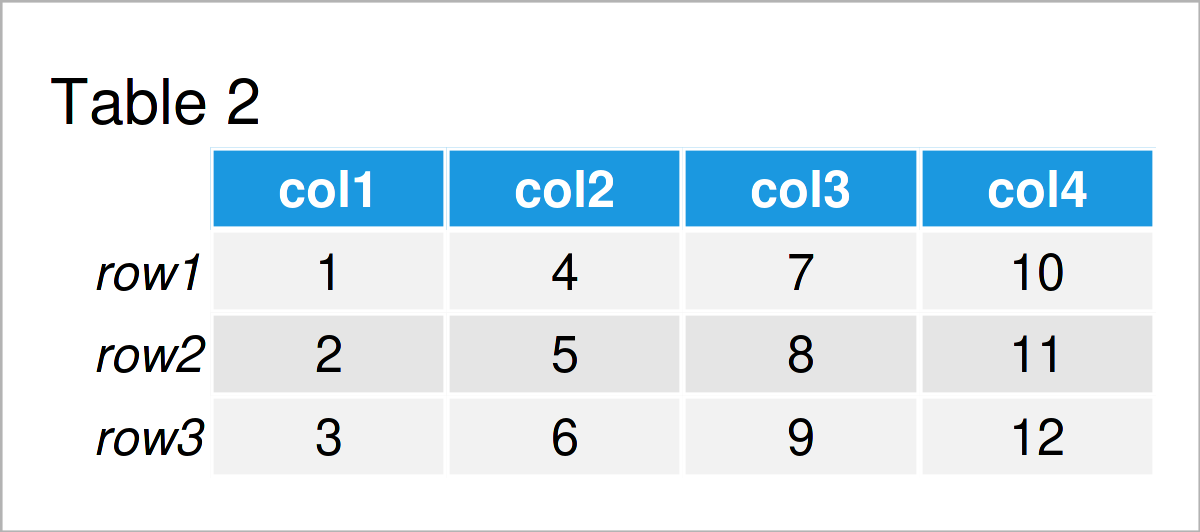# dimnames Function in R (2 Examples)

This article illustrates how to get and set the dimnames of a data object using the dimnames() function in R programming.

The article is structured as follows:

Let’s start right away…

## Creation of Example Data

I’ll use the following data as basement for this R programming tutorial:

```data <- matrix(1:12, ncol = 4)             # Create example matrix
data                                       # Print example matrix```Table 1 shows the structure of our example data – It has three rows and four columns.

In the examples of this tutorial, we’ll use a matrix object. However, please note that the dimnames function could be applied to other data types such as data frames or arrays as well.

## Example 1: Set Dimnames of Data object

In this example, I’ll show how to define the names of the dimensions of a data set.

As first step, we have to define a list object that contains the new row and column names that we want to assign as new dimnames:

```my_dimnames <- list(paste0("row", 1:3),    # Create list of dimnames
paste0("col", 1:4))
my_dimnames                                # Print list of dimnames
# []
#  "row1" "row2" "row3"
#
# []
#  "col1" "col2" "col3" "col4"```

Have a look at the previous output of the RStudio console. As you can see, we have specified three new row names and four new column names. Note that the number of row and column names has to be equal to the number of rows and columns in our data set.

Next, we can apply the dimnames function to our data set to change the row and column names of these data:

```dimnames(data) <- my_dimnames              # Assign dimnames to matrix
data                                       # Print updated matrix```As shown in Table 2, the previous syntax has created a new version of our matrix. This updated matrix has new row and column names (i.e. new dimnames).

## Example 2: Retrieve Dimnames of Data object

The dimnames function can also be used to print the dimnames of a data set.

In this example, I’ll show how to return the dimnames of the updated data matrix that we have created in Example 1.

For this, we simply have to apply the dimnames function to our data matrix as shown below:

```dimnames(data)                             # Return dimnames of matrix
# []
#  "row1" "row2" "row3"
#
# []
#  "col1" "col2" "col3" "col4"```

The previous output of the RStudio console shows the dimnames of our matrix object.

## Video & Further Resources

Have a look at the following video on my YouTube channel. In the video, I’m explaining the R code of the present tutorial.

Furthermore, you may want to read some of the other tutorials on my website. Some tutorials that are related to the application of the dimnames() function are listed below:

In this tutorial you have learned how to retrieve and specify the dimnames of a data object using the dimnames() function in the R programming language. In case you have additional questions, don’t hesitate to let me know in the comments below.

Subscribe to the Statistics Globe Newsletter